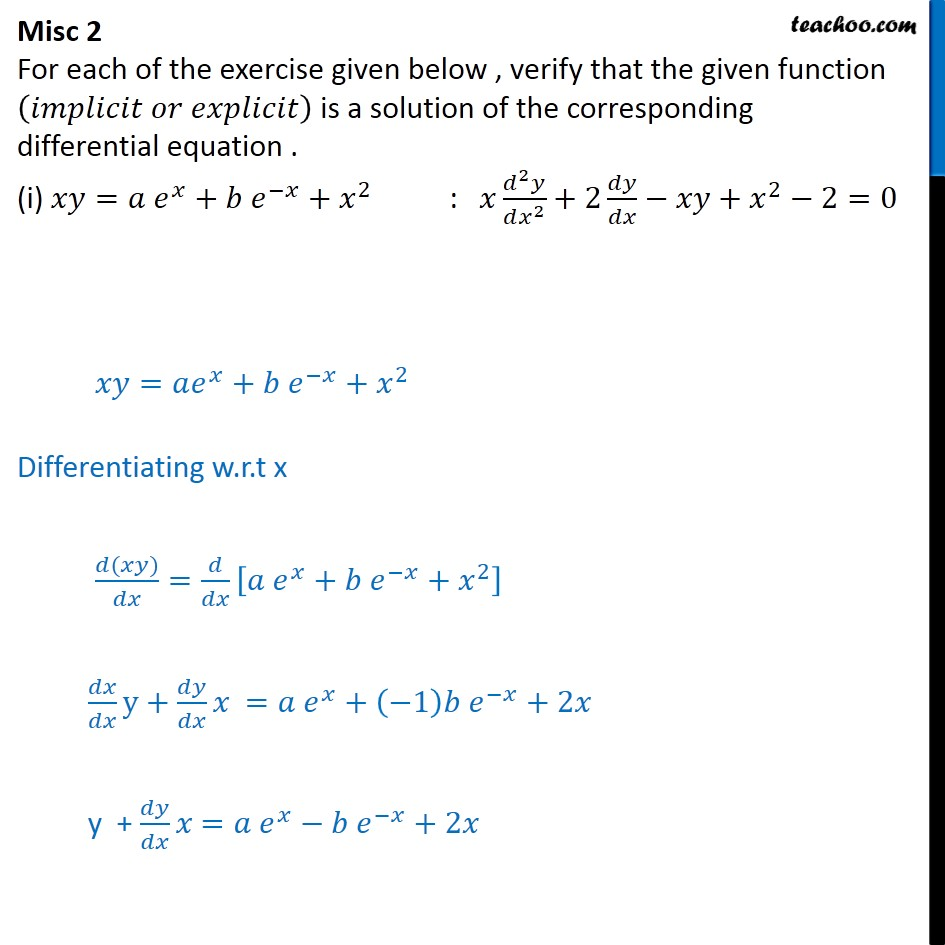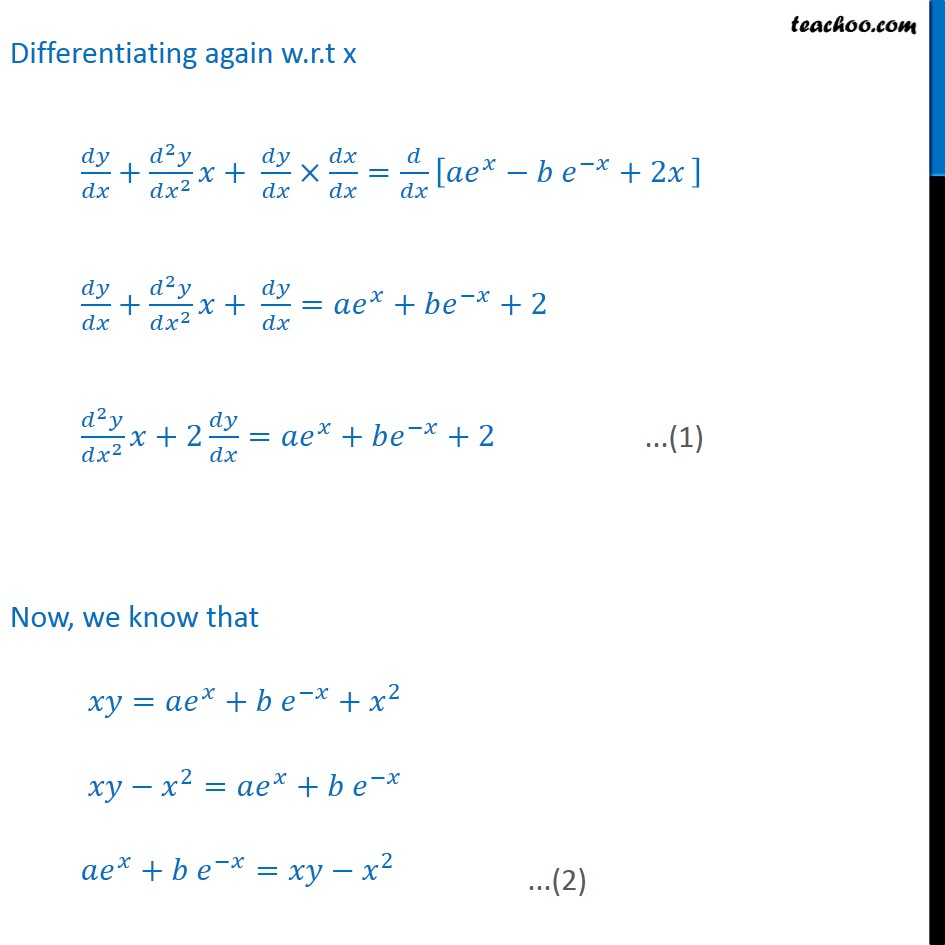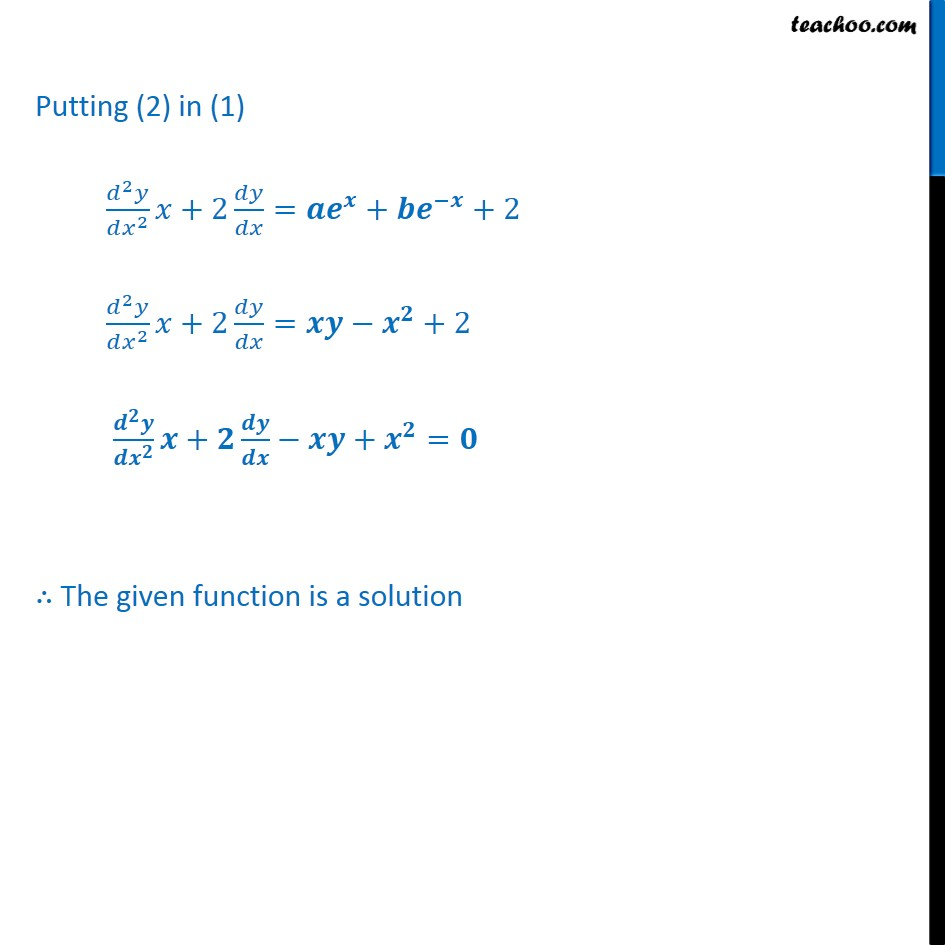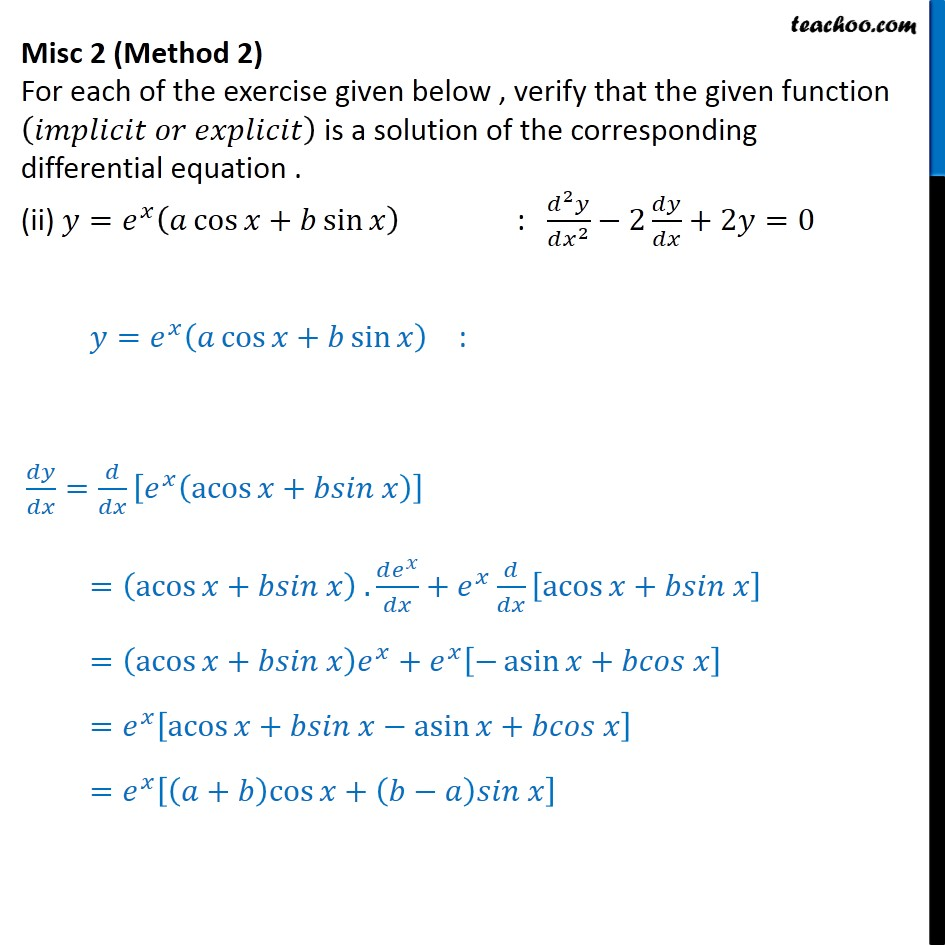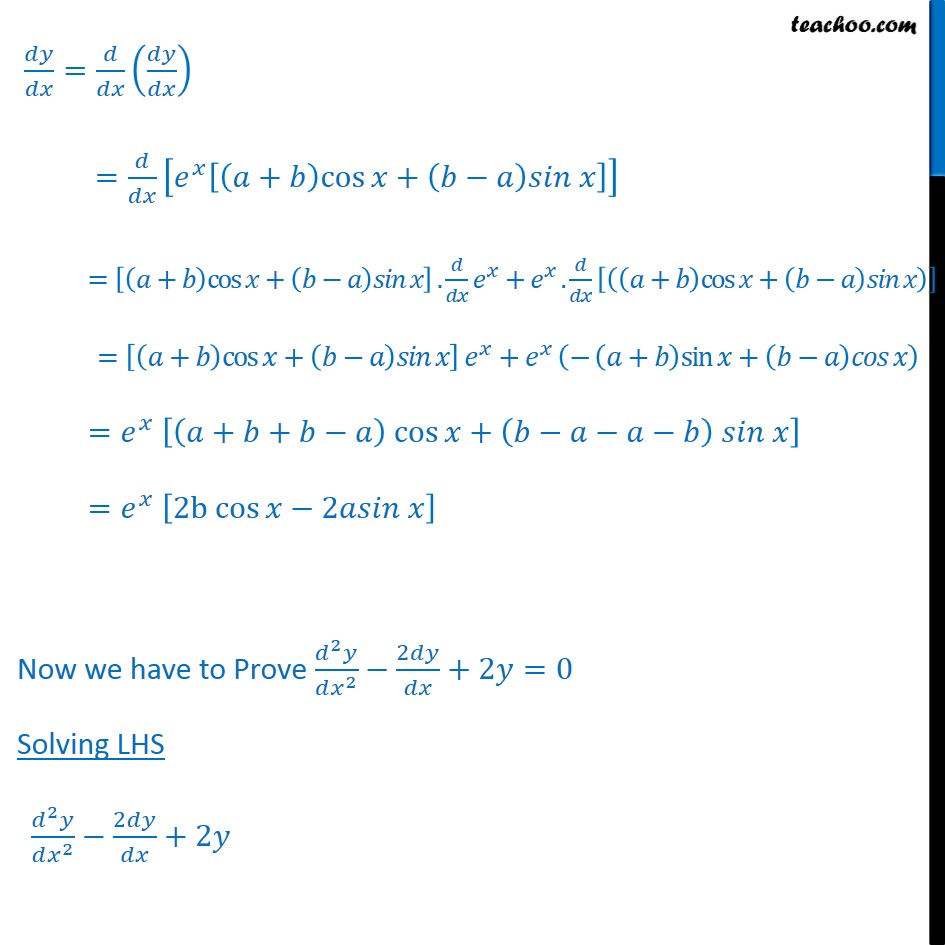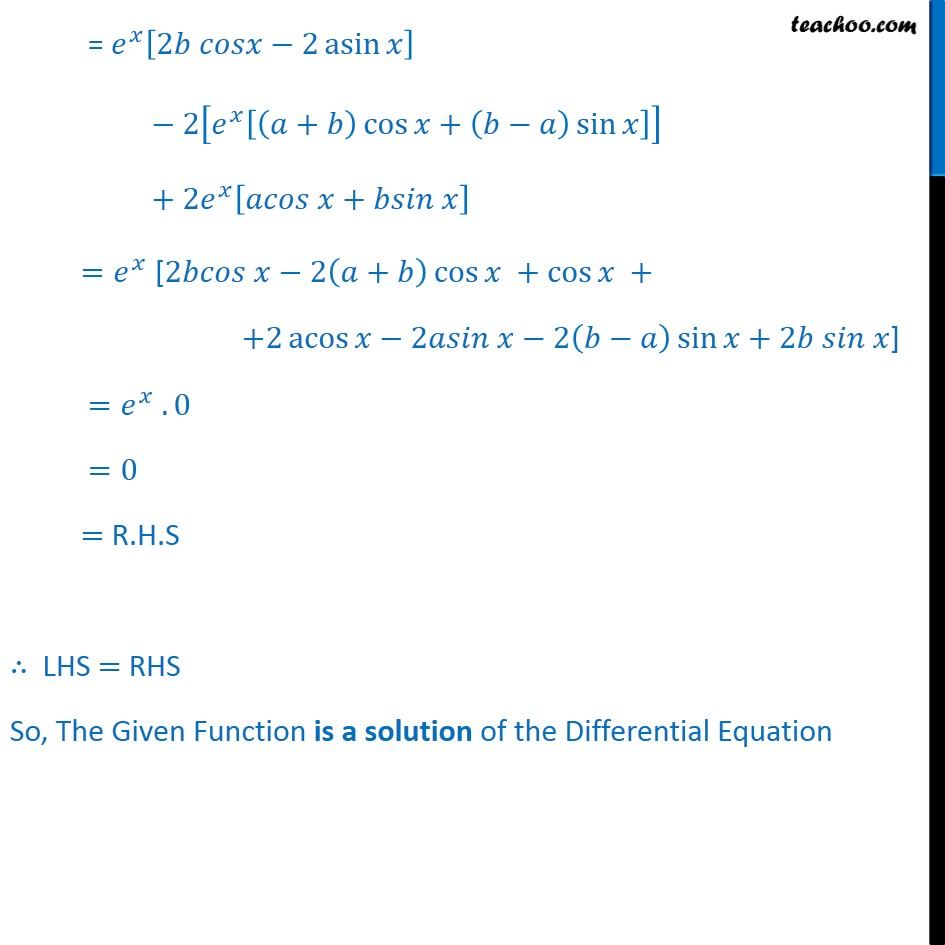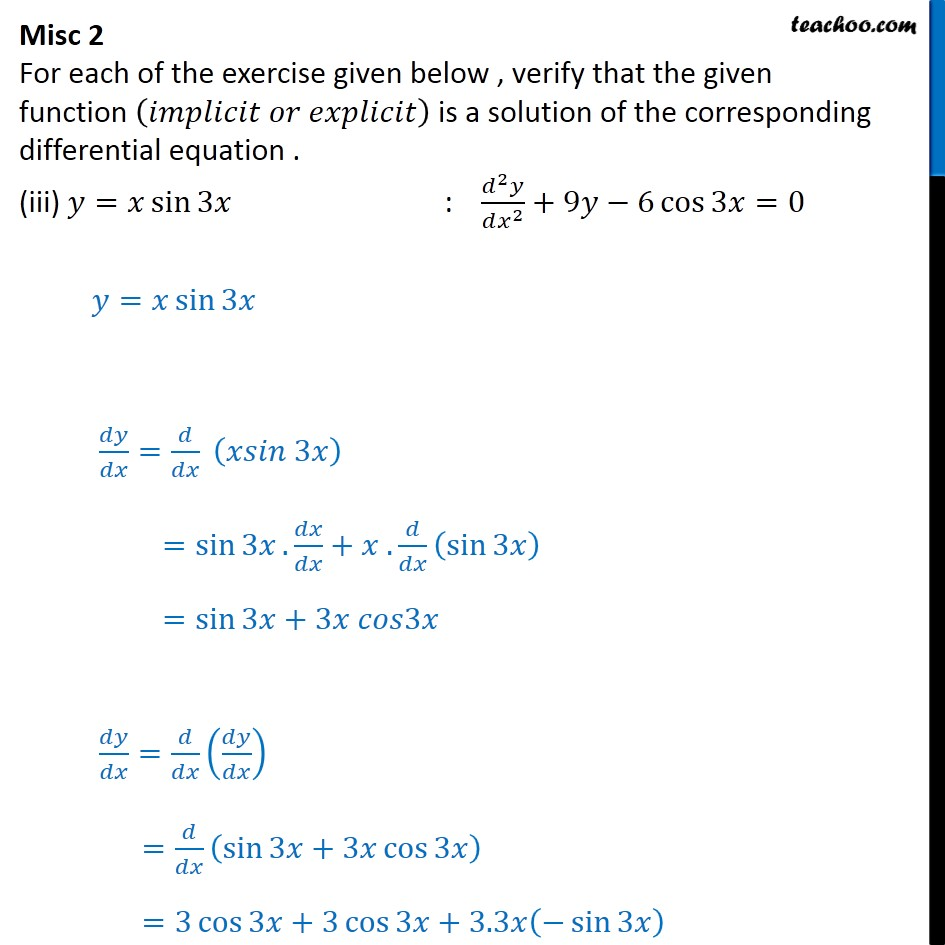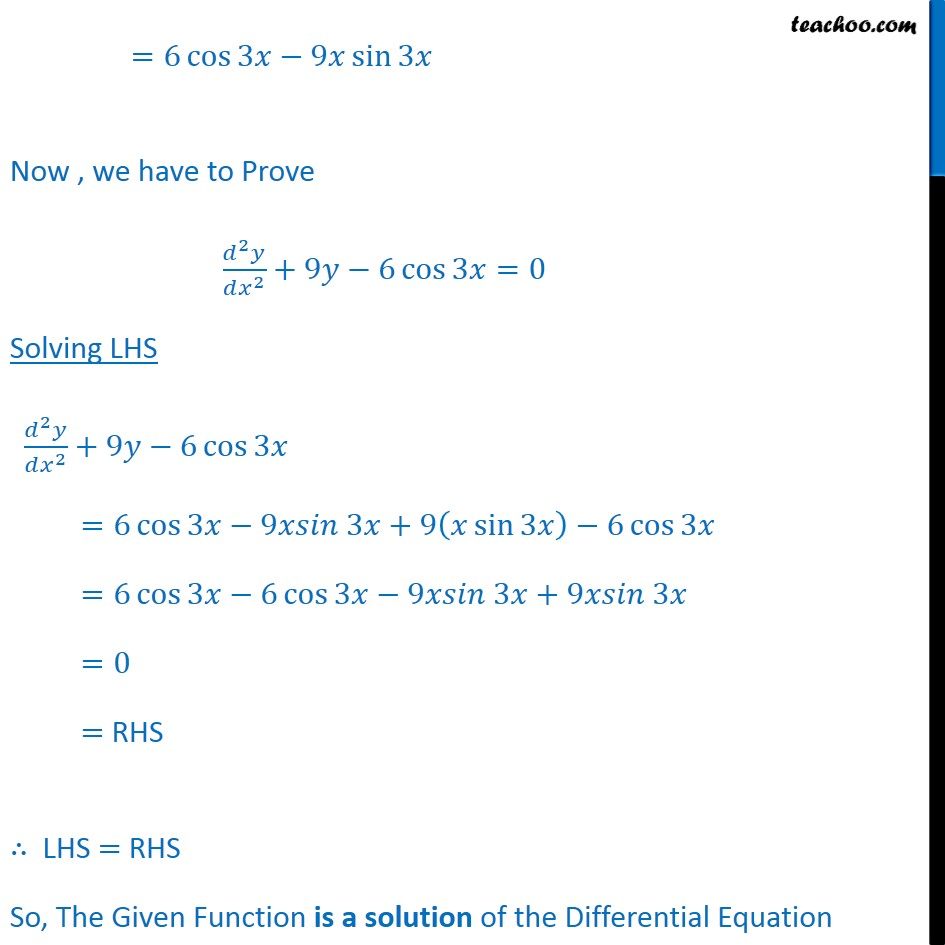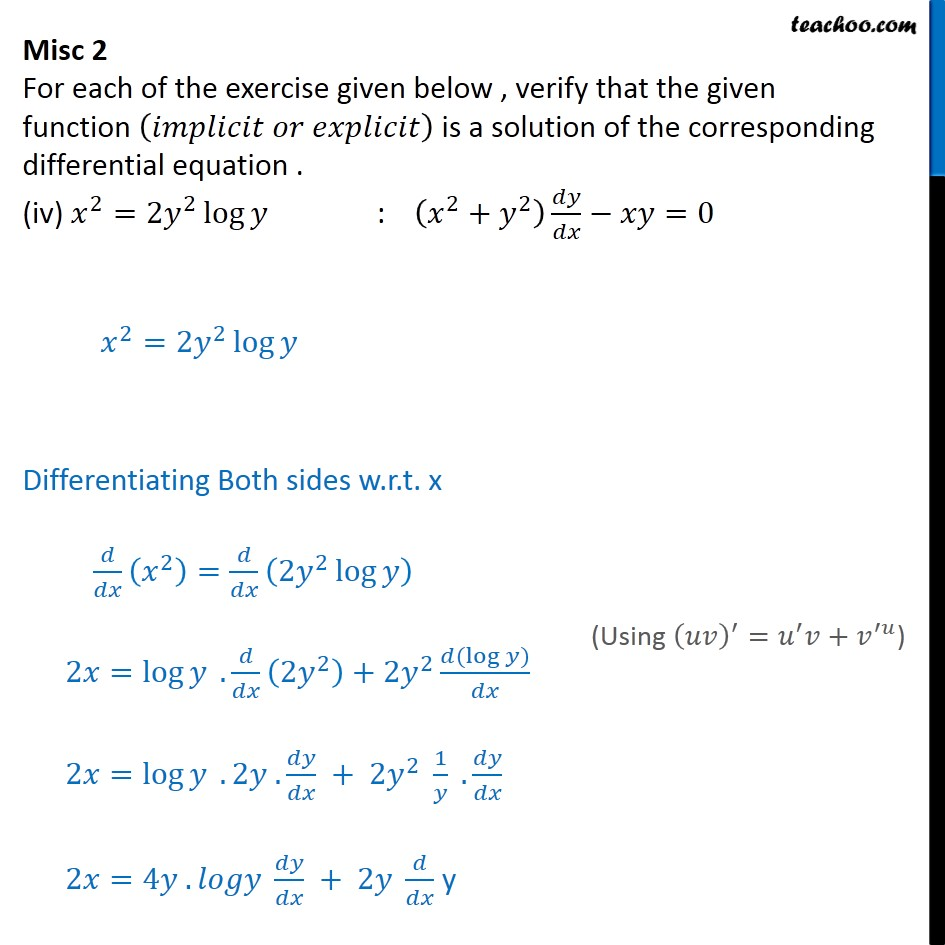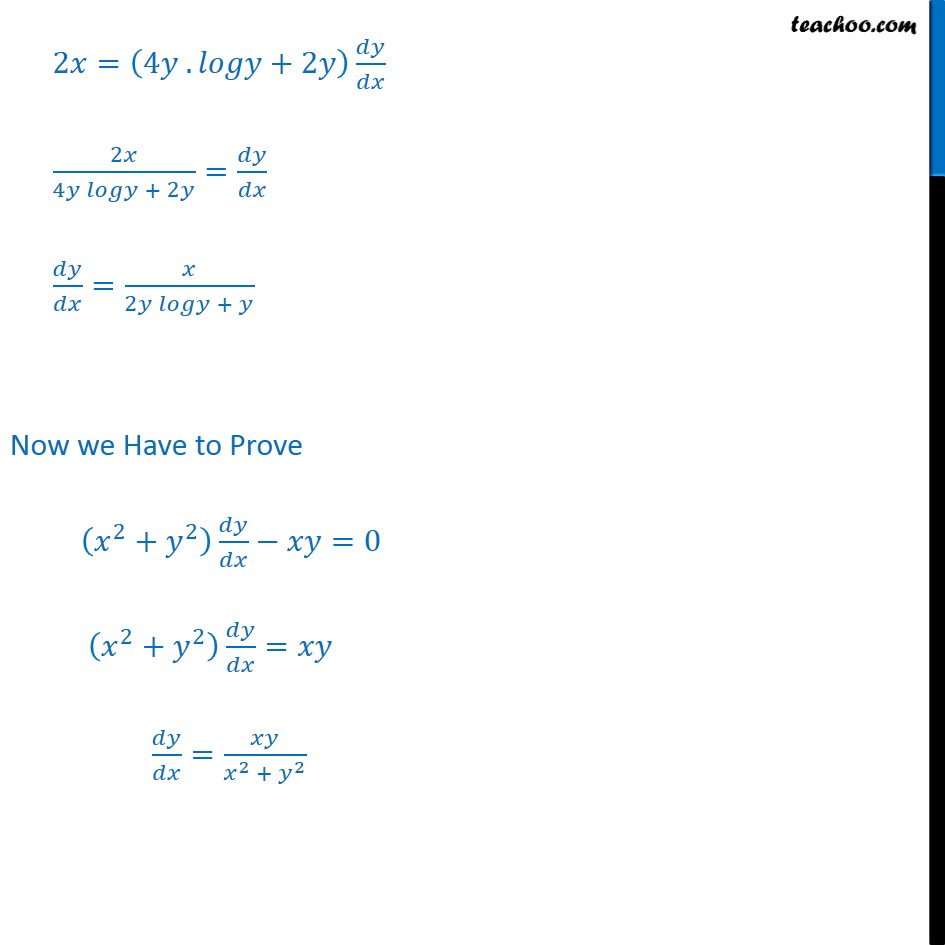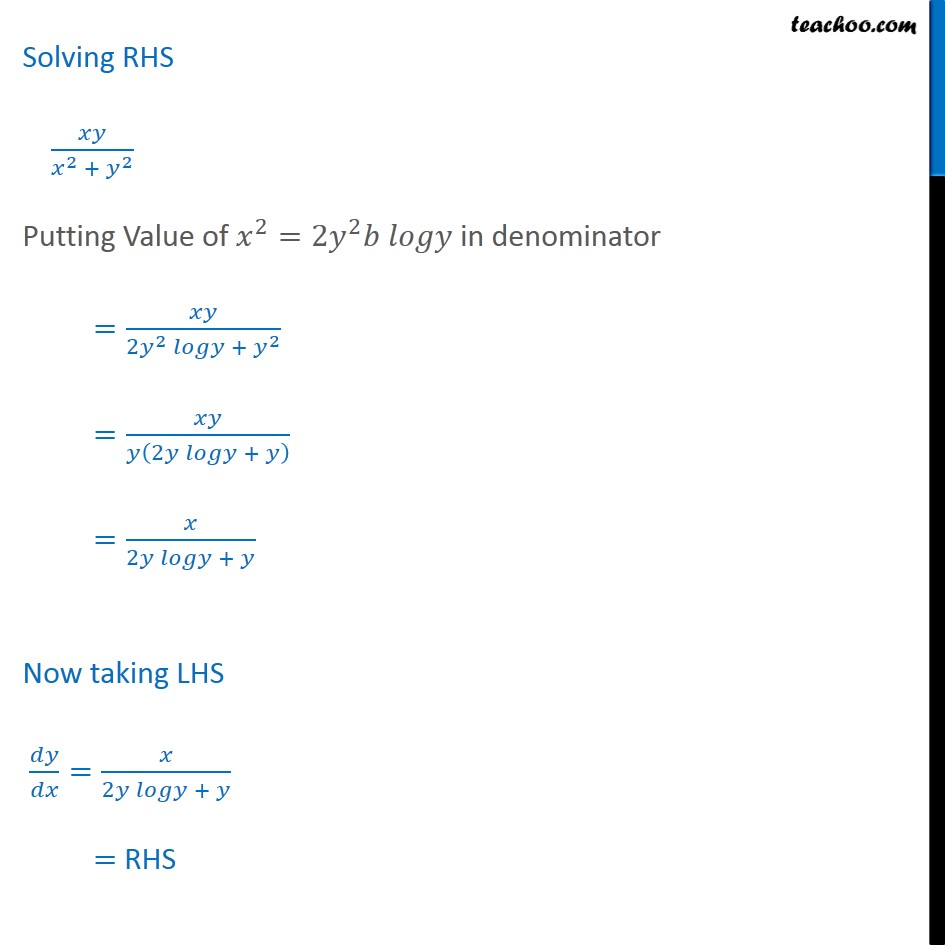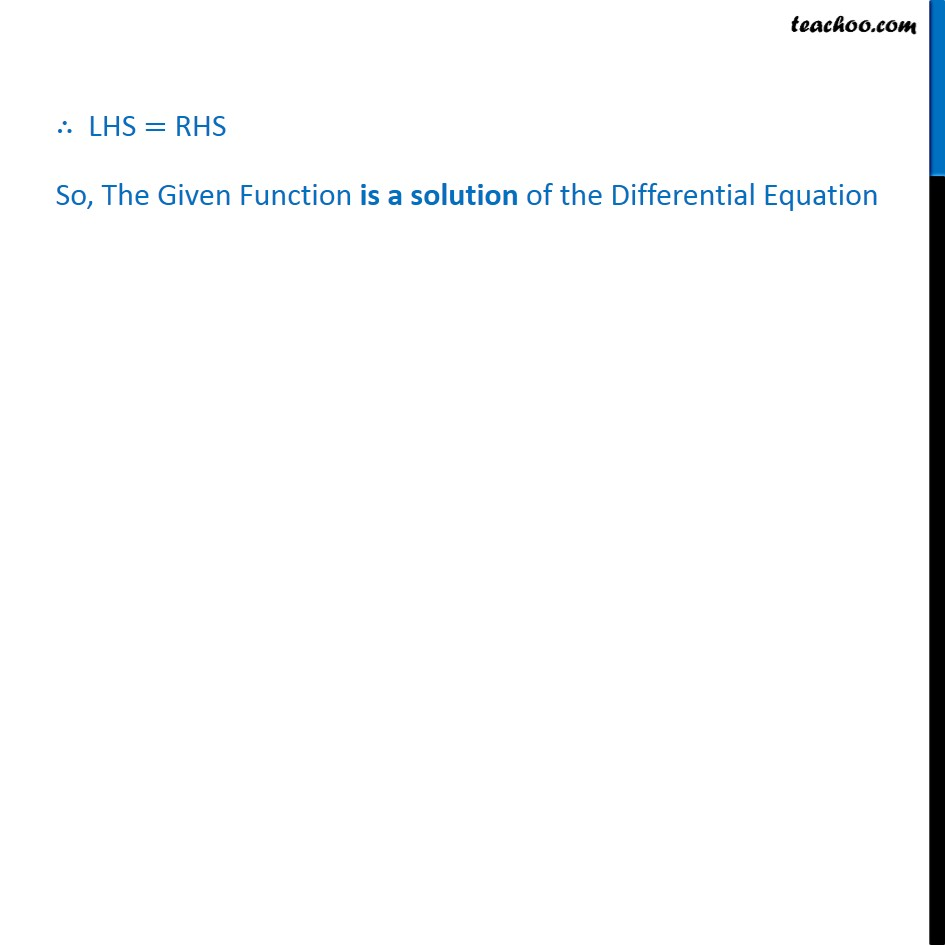1. Chapter 9 Class 12 Differential Equations
2. Serial order wise
3. Miscellaneous

Transcript

Misc 2 For each of the exercise given below , verify that the given function (𝑖𝑚𝑝𝑙𝑖𝑐𝑖𝑡 𝑜𝑟 𝑒𝑥𝑝𝑙𝑖𝑐𝑖𝑡) is a solution of the corresponding differential equation . (i) 𝑥𝑦=𝑎 𝑒^𝑥+𝑏 𝑒^(−𝑥)+𝑥^2 : 𝑥 (𝑑^2 𝑦)/(𝑑𝑥^2 )+2 𝑑𝑦/𝑑𝑥−𝑥𝑦+𝑥^2−2=0 𝑥𝑦=𝑎𝑒^𝑥+𝑏 𝑒^(−𝑥)+𝑥^2 Differentiating w.r.t x (𝑑(𝑥𝑦))/𝑑𝑥=𝑑/𝑑𝑥 [𝑎 𝑒^𝑥+𝑏 𝑒^(−𝑥)+𝑥^2 ] 𝑑𝑥/𝑑𝑥 y+𝑑𝑦/𝑑𝑥 𝑥 =𝑎〖 𝑒〗^𝑥+(−1)𝑏 𝑒^(−𝑥)+2𝑥 y + 𝑑𝑦/𝑑𝑥 𝑥 =𝑎〖 𝑒〗^𝑥−𝑏 𝑒^(−𝑥)+2𝑥 Differentiating again w.r.t x 𝑑𝑦/𝑑𝑥+ (𝑑^2 𝑦)/(𝑑𝑥^2 ) 𝑥+ 𝑑𝑦/𝑑𝑥×𝑑𝑥/𝑑𝑥=𝑑/𝑑𝑥 [𝑎𝑒^𝑥−𝑏 𝑒^(−𝑥)+2𝑥" " ] 𝑑𝑦/𝑑𝑥+ (𝑑^2 𝑦)/(𝑑𝑥^2 ) 𝑥+ 𝑑𝑦/𝑑𝑥=𝑎𝑒^𝑥+𝑏𝑒^(−𝑥)+2 (𝑑^2 𝑦)/(𝑑𝑥^2 ) 𝑥+2 𝑑𝑦/𝑑𝑥=𝑎𝑒^𝑥+𝑏𝑒^(−𝑥)+2 Now, we know that 𝑥𝑦=𝑎𝑒^𝑥+𝑏 𝑒^(−𝑥)+𝑥^2 𝑥𝑦−𝑥^2=𝑎𝑒^𝑥+𝑏 𝑒^(−𝑥) 𝑎𝑒^𝑥+𝑏 𝑒^(−𝑥)=𝑥𝑦−𝑥^2 Putting (2) in (1) (𝑑^2 𝑦)/(𝑑𝑥^2 ) 𝑥+2 𝑑𝑦/𝑑𝑥=𝒂𝒆^𝒙+𝒃𝒆^(−𝒙)+2 (𝑑^2 𝑦)/(𝑑𝑥^2 ) 𝑥+2 𝑑𝑦/𝑑𝑥=𝒙𝒚−𝒙^𝟐+2 (𝒅^𝟐 𝒚)/(𝒅𝒙^𝟐 ) 𝒙+𝟐 𝒅𝒚/𝒅𝒙−𝒙𝒚+𝒙^𝟐=𝟎 ∴ The given function is a solution Misc 2 (Method 2) For each of the exercise given below , verify that the given function (𝑖𝑚𝑝𝑙𝑖𝑐𝑖𝑡 𝑜𝑟 𝑒𝑥𝑝𝑙𝑖𝑐𝑖𝑡) is a solution of the corresponding differential equation . (ii) 𝑦=𝑒^𝑥 (𝑎 cos⁡〖𝑥+𝑏 sin⁡𝑥 〗 ) : (𝑑^2 𝑦)/(𝑑𝑥^2 )−2 𝑑𝑦/𝑑𝑥+2𝑦=0 𝑦=𝑒^𝑥 (𝑎 cos⁡〖𝑥+𝑏 sin⁡𝑥 〗 ) : 𝑑𝑦/𝑑𝑥=𝑑/𝑑𝑥 [𝑒^𝑥 (acos⁡〖𝑥+𝑏𝑠𝑖𝑛 𝑥〗 )] =(acos⁡〖𝑥+𝑏𝑠𝑖𝑛 𝑥〗 ) . (𝑑𝑒^𝑥)/𝑑𝑥+𝑒^𝑥 𝑑/𝑑𝑥 [acos⁡〖𝑥+𝑏𝑠𝑖𝑛 𝑥〗 ] =(acos⁡〖𝑥+𝑏𝑠𝑖𝑛 𝑥〗 ) 𝑒^𝑥+𝑒^𝑥 [−asin⁡〖𝑥+𝑏𝑐𝑜𝑠 𝑥〗 ] =𝑒^𝑥 [acos⁡〖𝑥+𝑏𝑠𝑖𝑛 𝑥〗−asin⁡〖𝑥+𝑏𝑐𝑜𝑠 𝑥〗 ] =𝑒^𝑥 [(𝑎+𝑏)cos⁡〖𝑥+(𝑏−𝑎)𝑠𝑖𝑛 𝑥〗 ] 𝑑𝑦/𝑑𝑥=𝑑/𝑑𝑥 (𝑑𝑦/𝑑𝑥) =𝑑/𝑑𝑥 [𝑒^𝑥 [(𝑎+𝑏)cos⁡〖𝑥+(𝑏−𝑎)𝑠𝑖𝑛 𝑥〗 ]] =[(𝑎+𝑏)cos⁡〖𝑥+(𝑏−𝑎)𝑠𝑖𝑛 𝑥〗 ] . 𝑑/𝑑𝑥 𝑒^𝑥+𝑒^𝑥 . 𝑑/𝑑𝑥 [((𝑎+𝑏)cos⁡〖𝑥+(𝑏−𝑎)𝑠𝑖𝑛 𝑥〗 )] =[(𝑎+𝑏)cos⁡〖𝑥+(𝑏−𝑎)𝑠𝑖𝑛 𝑥〗 ] 𝑒^𝑥+𝑒^𝑥 (−(𝑎+𝑏)sin⁡〖𝑥+(𝑏−𝑎)𝑐𝑜𝑠 𝑥〗 ) =𝑒^𝑥 [〖(𝑎+𝑏+𝑏−𝑎) cos〗⁡〖𝑥+(𝑏−𝑎−𝑎−𝑏) 𝑠𝑖𝑛 𝑥〗 ] =𝑒^𝑥 [〖2b cos〗⁡〖𝑥−2𝑎𝑠𝑖𝑛 𝑥〗 ] Now we have to Prove (𝑑^2 𝑦)/(𝑑𝑥^2 )−2𝑑𝑦/𝑑𝑥+2𝑦=0 Solving LHS (𝑑^2 𝑦)/(𝑑𝑥^2 )−2𝑑𝑦/𝑑𝑥+2𝑦 = 𝑒^𝑥 [2𝑏 𝑐𝑜𝑠𝑥−2 asin⁡𝑥 ] − 2[𝑒^𝑥 [(𝑎+𝑏) cos⁡𝑥+(𝑏−𝑎) sin⁡𝑥 ]] + 2𝑒^𝑥 [𝑎𝑐𝑜𝑠 𝑥+𝑏𝑠𝑖𝑛 𝑥] =𝑒^𝑥 [2𝑏𝑐𝑜𝑠 𝑥−2(𝑎+𝑏) cos⁡〖𝑥 〗+cos⁡〖𝑥 〗+ +2 acos⁡〖𝑥−2𝑎𝑠𝑖𝑛 𝑥〗−2(𝑏−𝑎) sin⁡〖𝑥+2𝑏 𝑠𝑖𝑛 𝑥〗] =𝑒^𝑥 . 0 =0 = R.H.S ∴ LHS = RHS So, The Given Function is a solution of the Differential Equation Misc 2 For each of the exercise given below , verify that the given function (𝑖𝑚𝑝𝑙𝑖𝑐𝑖𝑡 𝑜𝑟 𝑒𝑥𝑝𝑙𝑖𝑐𝑖𝑡) is a solution of the corresponding differential equation . (iii) 𝑦=𝑥 sin⁡3𝑥 : (𝑑^2 𝑦)/(𝑑𝑥^2 )+9𝑦−6 cos⁡〖3𝑥=0〗 𝑦=𝑥 sin⁡3𝑥 𝑑𝑦/𝑑𝑥=𝑑/𝑑𝑥 (𝑥𝑠𝑖𝑛 3𝑥) =sin⁡3𝑥. 𝑑𝑥/𝑑𝑥+𝑥 . 𝑑/𝑑𝑥 (sin⁡3𝑥 ) =sin⁡3𝑥+3𝑥 𝑐𝑜𝑠3𝑥 𝑑𝑦/𝑑𝑥=𝑑/𝑑𝑥 (𝑑𝑦/𝑑𝑥) =𝑑/𝑑𝑥 (sin⁡〖3𝑥+3𝑥 cos⁡3𝑥 〗 ) =3 cos⁡〖3𝑥+3 cos⁡〖3𝑥+3.3𝑥(−sin⁡3𝑥 )〗 〗 =6 cos⁡〖3𝑥−9𝑥 sin⁡3𝑥 〗 Now , we have to Prove (𝑑^2 𝑦)/(𝑑𝑥^2 )+9𝑦−6 cos⁡〖3𝑥=0〗 Solving LHS (𝑑^2 𝑦)/(𝑑𝑥^2 )+9𝑦−6 cos⁡3𝑥 =6 cos⁡3𝑥−9𝑥𝑠𝑖𝑛 3𝑥+9(𝑥 sin⁡3𝑥 )−6 cos⁡3𝑥 =6 cos⁡3𝑥−6 cos⁡3𝑥−9𝑥𝑠𝑖𝑛 3𝑥+9𝑥𝑠𝑖𝑛 3𝑥 =0 = RHS ∴ LHS = RHS So, The Given Function is a solution of the Differential Equation Misc 2 For each of the exercise given below , verify that the given function (𝑖𝑚𝑝𝑙𝑖𝑐𝑖𝑡 𝑜𝑟 𝑒𝑥𝑝𝑙𝑖𝑐𝑖𝑡) is a solution of the corresponding differential equation . (iv) 𝑥^2=2𝑦^2 log⁡𝑦 : (𝑥^2+𝑦^2 ) 𝑑𝑦/𝑑𝑥−𝑥𝑦=0 𝑥^2=2𝑦^2 log⁡𝑦 Differentiating Both sides w.r.t. x 𝑑/𝑑𝑥 (𝑥^2 )=𝑑/𝑑𝑥 (2𝑦^2 log⁡𝑦 ) 2𝑥=log⁡𝑦 . 𝑑/𝑑𝑥 (2𝑦^2 )+2𝑦^2 (𝑑(log⁡〖𝑦)〗)/𝑑𝑥 2𝑥=log⁡𝑦 . 2𝑦 . 𝑑𝑦/𝑑𝑥 + 2𝑦^2 1/𝑦 . 𝑑𝑦/𝑑𝑥 2𝑥=4𝑦 . 𝑙𝑜𝑔𝑦 𝑑𝑦/𝑑𝑥 + 2𝑦 𝑑/𝑑𝑥 y 2𝑥=(4𝑦 . 𝑙𝑜𝑔𝑦+2𝑦) 𝑑𝑦/𝑑𝑥 2𝑥/(4𝑦 𝑙𝑜𝑔𝑦 + 2𝑦)=𝑑𝑦/𝑑𝑥 𝑑𝑦/𝑑𝑥=𝑥/(2𝑦 𝑙𝑜𝑔𝑦 + 𝑦) Now we Have to Prove (𝑥^2+𝑦^2 ) 𝑑𝑦/𝑑𝑥−𝑥𝑦=0 (𝑥^2+𝑦^2 ) 𝑑𝑦/𝑑𝑥=𝑥𝑦 𝑑𝑦/𝑑𝑥=𝑥𝑦/(𝑥^2 +〖 𝑦〗^2 ) Solving RHS 𝑥𝑦/(𝑥^2 + 𝑦^2 ) Putting Value of 𝑥^2=2𝑦^2 𝑏 𝑙𝑜𝑔𝑦 in denominator =𝑥𝑦/(2𝑦^2 𝑙𝑜𝑔𝑦 +〖 𝑦〗^2 ) =𝑥𝑦/𝑦(2𝑦 𝑙𝑜𝑔𝑦 + 𝑦) =𝑥/(2𝑦 𝑙𝑜𝑔𝑦 + 𝑦) Now taking LHS 𝑑𝑦/𝑑𝑥=𝑥/(2𝑦 𝑙𝑜𝑔𝑦 + 𝑦) = RHS ∴ LHS = RHS So, The Given Function is a solution of the Differential Equation

Miscellaneous